Definition: measures of central tendency from Dictionary of Psychological Testing, Assessment and Treatment

summary statistics which divide the data into two halves (i.e. half of the data fall below the figure produced, half above). This is an exact division in the case of the median, but can be more approximate for the mean and mode.

Summary Article: Measures of Central Tendency
From Encyclopedia of Measurement and Statistics

Measures of central tendency are measures of the location of the center or middle of a distribution. However, the definition of “center” or “middle” is deliberately left broad, such that the term central tendency can refer to a wide variety of measures. The three most common measures of central tendency are the mode, the mean, and the median.

Mode

The mode for a collection of data values is the data value that occurs most frequently (if there is one). Suppose the average number of colds in a family of six in a calendar year is as presented in Table 1.

Then, the mode is 1 because more family members (i.e., n = 2) caught one cold than any other number of colds. Thus, 1 is the most frequently occurring value. If two values occur the same number of times and more often than the others, then the data set is said to be bimodal. The data set is multimodal if there are more than two values that occur with the same greatest frequency. The mode is applicable to qualitative as well as quantitative data.

With continuous data, such as the time patients spend waiting at a particular doctor's office, which can be measured to many decimals, the frequency of each value is most commonly 1 because no two scores will be identical. Consequently, for continuous data, the mode typically is computed from a grouped frequency distribution. The grouped frequency distribution in Table 2 shows a grouped frequency distribution for the waiting times of 20 patients. Because the interval with the highest frequency is 30 - <40 minutes, the mode is the middle of that interval (i.e., 35 minutes).

Table 1: Number of Colds in a Selected Family
Family Member Frequency
Father 5
Mother 4
First Son 1
Second Son 2
First Daughter 1
Second Daughter 3
Table 2: Grouped Frequency Distribution
Range Frequency
0 − <10 2
10 − <20 2
20 − <30 3
30 − <40 7
40 − <50 3
50 − <60 2
60 − <70 1
Mean
Arithmetic Mean

The arithmetic mean, or average, is the most common measure of central tendency. Given a collection of data values, the mean of these data is simply the arithmetic average of these data values. That is, the mean is the sum of observations divided by the number of observations. If we use the following notation:

x is the variable for which we have data (e.g, test scores),

n is the number of sample observations (sample size),

x1 is the first sample observation (first test score),

x2 is the second sample observation (second test score),

xn is the nth (last) sample observation (last test score),

then the sample mean of a sample x1, x2,…, xn is denoted by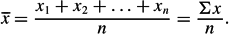To find the average number of colds in the family presented earlier, we compute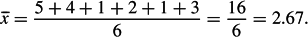The arithmetic mean is not the only “mean” available. Indeed, there is another kind of mean that is called the geometric mean, which is explained below. However, the arithmetic mean is by far the most commonly used. Consequently, when the term mean is used, one can assume that it is the arithmetic mean.

Weighted Mean

The weighted mean of a set of measurements x1, x2,…, xn with relative weights w1, w2,…, wn is given by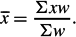The weighted mean has many applications. In effect, it is used to approximate the mean of data grouped in a frequency distribution. In order to approximate the mean waiting time of the 20 patients presented above, the class mark is used to represent the waiting time of each person falling within that class. A weighted mean is then calculated, where the xs are the class marks and the weights are the corresponding class frequencies, as in Table 3.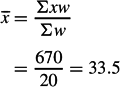Thus, the weighted mean is 33.5 minutes. The mean has two important properties. First, the sum of the deviations of all scores in the distribution from the mean is zero. Second, the sum of squares of deviations about the mean is smaller than the sum of squares of deviations about any other value. Consequently, the mean is the measure of central tendency in the least squares sense inasmuch as the sum of the squared deviations is a minimum.

Table 3: Computation of Weighted Mean
Waiting Time Class Mark(x) Frequency(w) x·w
0 - <10 5 2 10
10.-.<20 15 2 30
20.-.<30 25 3 75
30.-.<40 35 7 245
40.-.<50 45 3 135
50.-.<60 55 2 110
60.-.<70 65 1 65
Trimean

The trimean is another measure of central tendency. It is computed by adding the 25th percentile plus twice the 50th percentile plus the 75th percentile, and then dividing by four. The 25th, 50th, and 75th percentile of the cold data set is 1, 2.5, and 4.25, respectively. Therefore, the trimean is computed as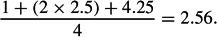The trimean value of 2.56 is close to the arithmetic mean value of 2.67. The trimean has logical appeal as a measure of central tendency. However, it is rarely used.

Trimmed Mean

The trimmed mean is computed by discarding a certain percentage of the lowest and the highest scores in a ranked (i.e., ordered) set of data and then computing the mean of the remaining scores. For example, a mean trimmed 50% is computed by discarding the highest and lowest 25% of the scores and taking the mean of the remaining scores. The mean trimmed 0% provides the arithmetic mean. Trimmed means are used in certain sporting events (e.g., ice skating, gymnastics) to judge competitors' levels of performance and to prevent the effects of extreme ratings possibly caused by biased judges. Before scores are discarded, the analyst must first rank the data. For the cold data, the mean trimmed 33% would result in the highest value (i.e., 5) and lowest value (i.e., 1) being discarded, resulting in the following trimmed mean: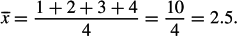Geometric Mean

The geometric mean of n numbers is obtained by multiplying all of them together, and then taking the nth root of them. In other words, the geometric mean is the nth root of the product of the n scores in the dataset. Thus, the geometric mean of the cold data— 5, 4, 1, 2, 1, and 3—is the sixth root of 5 × 4 × 1 × 2 × 1 × 3, which is the sixth root of 120 (because there are six numbers), which equals 2.22. The formula can be written as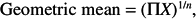where πX means to take the product of all the values of X, and the superscript value (i.e., 1/n) indicates the nth root. The geometric mean can also be computed by

1. computing the logarithm of each number,

2. computing the arithmetic mean of the logarithms,

3. raising the base used to take the logarithms to the arithmetic mean.

Thus, if the natural logarithm (i.e., Ln) is used, then raising this base would necessitate use of the exponent. For the cold data, the computation would be as in Table 4.

The base of natural logarithms is 2.718. The expression EXP[0.7979] means that 2.718 is raised to the 0.7979th power. Ln(X) is the natural log of X.

An identical result can be obtained by using logs base 10 as shown in Table 5.

If any one of the scores is zero, then the geometric mean is zero. If any scores are negative, then the geometric mean is meaningless. The geometric mean is an appropriate measure to use for averaging rates. However, it is one of the least used measures of central tendency.

Table 4: Computation of Geometric Mean Using Natural Logarithms
Family Members Frequency(x) Ln(X)
Father 5 1.6094
Mother 4 1.3863
First Son 1 0.0000
Second Son 2 0.6931
First Daughter 1 0.0000
Second Daughter 3 1.0986
Arithmetic mean 0.7979
Exponential EXP [0.7979] = 2.22
Geometric mean 2.22
Table 5: Computation of Geometric Mean Using Base 10 Logarithms
Family Members Frequency(x) Ln(X)
Father 5 0.6990
Mother 4 0.6021
First Son 1 0.0000
Second Son 2 0.3010
First Daughter 1 0.0000
Second Daughter 3 0.4771
Arithmetic mean 0.3465
Exponential 100.3465 = 2.22
Geometric mean 2.22
Harmonic Mean

The harmonic mean is the mean of n numbers expressed as the reciprocal of the arithmetic mean of the reciprocals of the numbers. The harmonic mean typically is used to take the mean of sample sizes. For the cold data, the harmonic mean is defined as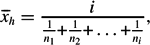where i is the number of scores from which the harmonic mean is computed. For the cold data, the harmonic mean is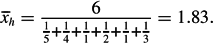This is less than the arithmetic mean of 2.67, the trimean of 2.56, and the geometric mean of 2.22.

Median

The median is the midpoint of a distribution such that the same number of scores is above the median as below it. In other words, the median is the 50th percentile. More specifically, the median for a collection of data values is the number that is exactly in the middle position of the list when the data are ranked (i.e., arranged in increasing order of magnitude). The formula for the median (Md) is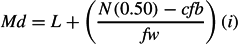where

• L is the lower limit of the interval within which the median lies,
• N is the number of cases in the distribution,
• cfb is the cumulative frequency in all intervals below the interval containing the median,
• fw is the frequency of cases within the interval containing the median,
• i is the interval size.

In order to compute the median for the cold data, the first step is to rank the data: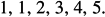Because there are six numbers (i.e., an even number of data points), there are two middle numbers, namely, 2 and 3. Therefore, L = 1.5 (i.e., the lowest of the two middle numbers - 0.5). Also, N = 6 (number of observations), and cfb = 2 (i.e., the number of observations that lie below the lower limit of 1.5). Also, fw = 2 (i.e., the number of observations that are equal to the middle numbers) because the two middle numbers (i.e., 2 and 3) do not appear anywhere else in the data set. Finally, i = 2 (i.e., the highest middle number - the lowest middle number + 1 = 3 - 2 + 1 = 2). (Please note that i = 1 if the middle numbers are all the same.) Thus, the median is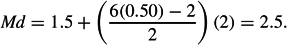Thus, the median cold is 2.5. When the number of observations is relatively small and the data are not grouped in class intervals—as is the case with the cold data—the median can be computed using the following steps:

1. Order the n observations from smallest to largest, including any repeated observations, so that every observation appears in the list.

2. Determine the location of the sample median, which is given by (n + 1)/2. Thus, for example, for a sample size of 5 (i.

3. e., n = 5), (n + 1)/2 = 3, and the median is represented by the third number in the series. For a sample size of 6, (n + 1)/2 = 3.5, and the median can be located somewhere between the third and fourth number in the series.

4. If the number of scores is odd, the median is the middle score. Consider the following ranked distribution of scores: 1, 3, 3, 5, 6, 7, 8, 8, 9. Because there are nine scores (i.

5. e., N = 9), the median is 6.

6. If the number of scores is even, the median is the average of the two middle values. Thus, because the cold data have an even number of scores, the two middle numbers are 2 and 3, and the median is the average of 2 and 3 (i.

7. e., the average of the third and fourth observations), which is 2.5.

It can be seen that the simpler method of calculating the median yielded exactly the same number as did using the more general formula. However, for relatively large sample sizes, the simpler formula can distort the true value of the median represented by the more general formula.

Computer Applications

When using SPSS, there are a few ways to compute measures of central tendency. The Frequencies command can be used to compute the mean, median, and mode. The Descriptives command can be used to compute the mean. The Explore command can be used to compute the median, mean, and trimmed mean. The Means command can be used to compute the median, mean, harmonic mean, and geometric mean. Finally, the Reports command can be used to compute the mean and median.

The SPSS output for the Frequencies command pertaining to the cold data set is presented in Figure 1.

The SPSS output for the Descriptives command pertaining to the cold data set is in Figure 2.

The SPSS output for the Explore command pertaining to the cold data set is in Figure 3.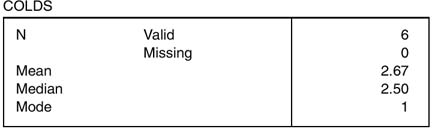Figure 1 Measures of Central Tendency Using Frequencies Command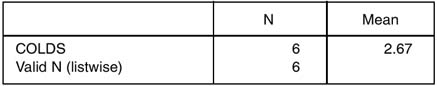Figure 2 Measures of Central Tendency Using Descriptives Command

The SPSS output for the Means command pertaining to the cold data set is in Figure 4.

The SPSS output for the Reports command pertaining to the cold data set is in Figure 5.

Comparisons of Measures of Central Tendency

To some extent, selection of the most appropriate measure of central tendency is dependent on the scale of measurement of the variable. Specifically, if the data are nominal, then only the mode is appropriate. If the data are ordinal, either the mode or the median may be appropriate. If the data are interval or ratio, the mode, median, or mean may be appropriate.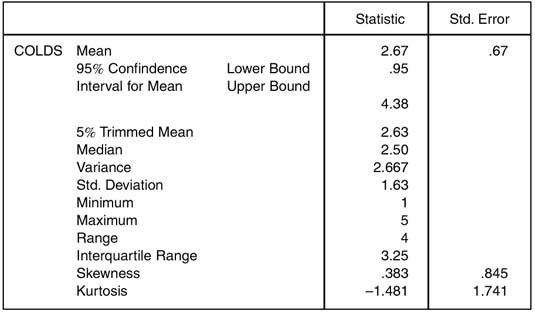Figure 3 Measures of Central Tendency Using Explore Command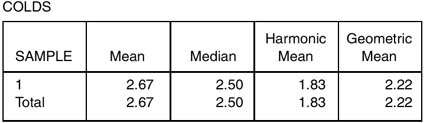Figure 4 Measures of Central Tendency Using Means Command

For distributions that are symmetrical and unimodal, the three major measures of central tendency (i.e., mean, median, mode) are all the same. When the distribution is symmetrical and bimodal, the mean and the median coincide, but two modes are present. The less symmetrical the distribution, the greater the differential between the mean, the median, and the mode. For skewed distributions, they can differ markedly. Specifically, in positively skewed distributions, the mean is higher than the median, whereas in negatively skewed distributions, the mean is lower than the median. Thus, comparing the mean and median can provide useful information about the level of skewness inherent in the distribution.

Of the eight measures of central tendency discussed, the mean is by far the most widely used because it takes every score into account, is the most efficient measure of central tendency for approximately symmetric (normal) distributions, and uses a simple formula. Also, because the mean requires that the differences between the various levels of the categories on any part of the distribution represent equal differences in the characteristic or trait measured (i.e., equal unit or interval/ratio scale), it can be manipulated mathematically in ways not appropriate to the median and mode. Thus, the mean is mathematically appealing, making it possible for researchers to develop statistical procedures for drawing inferences about means. However, the mean does have several disadvantages. In particular, the mean is sensitive to skewed data. It is also sensitive to outliers. Thus, the mean often is misleading in highly skewed distributions and is less efficient than other measures of central tendency when extreme scores are possible.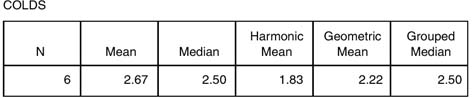Figure 5 Measures of Central Tendency Using Reports Command

The trimean is almost as resistant to extreme scores as is the median, and it is less subject to sampling fluctuations than the arithmetic mean in extremely skewed distributions. However, it is less efficient than the mean for normal distributions. The trimmed mean, which generally falls between the mean and the median, is less susceptible to the effects of extreme scores than the arithmetic mean and, in turn, is less susceptible to sampling fluctuation than the mean for extremely skewed distributions. However, like the trimean, the trimmed mean is less efficient than the mean for normal distributions. The geometric mean is less affected by extreme values than the arithmetic mean and is useful as a measure of central tendency for some positively skewed distributions. However, the geometric mean is rarely used because (a) it equals zero if any one of the scores is zero, regardless of how large the remaining scores are; (b) it is meaningless if any scores are negative; and (c) it is more difficult to compute than the arithmetic mean. The weighted mean does not use any of the actual scores in the distribution.

The median is useful because of its ease of interpretation and because it is more efficient than the mean in highly skewed distributions. That is, the median is not sensitive to skewed data. However, it does not take into account every score, relying only on the middle value(s) in an ordered set of data. Also, the median generally is less efficient than the mean, the trimean, and the trimmed mean. The mode can be informative, is easy to interpret, and is the only measure of central tendency that can be used with nominal data; however, it should almost never be used as the only measure of central tendency because it depends only on the most frequent observation and is highly susceptible to sampling fluctuations. Another disadvantage of the mode is that many distributions have more than one mode, thereby complicating interpretation. Also, the mode does not always exist.

Mean, Median, Mode

Means and medians graphical comparison applets: http://www.ruf.rice.edu/∼lane/stat_sim/descriptive/ and http://standards.nctm.org/document/eexamples/chap6/6.6/

• , , & (2005). SPSS for intermediate statistics: Use and interpretation (2nd ed.). Mahwah, NJ: Erlbaum.
• , , , & (2004). SPSS for basic statistics: Use and interpretation (2nd ed.). Mahwah, NJ: Erlbaum.

•Copyright © 2007 by SAGE Publications, Inc.

### Related Articles

##### Full text Article Measures of Central Tendency
Encyclopedia of Medical Decision Making

When physicians or clinical researchers encounter a sample of data, they often try to get an overall picture about the data before proceeding...

##### Full text Article Measures of Central Tendency
International Encyclopedia of Education

When trying to summarize a set of data, for example, the reading ability of a class of children, it can be useful to have a measure that tries...

##### Full text Article measures of central tendency
Collins Dictionary of Sociology

the different ways of conceptualizing the central or middle position of a group of observations, numbers, etc. There are three measures of...

See more from Credo